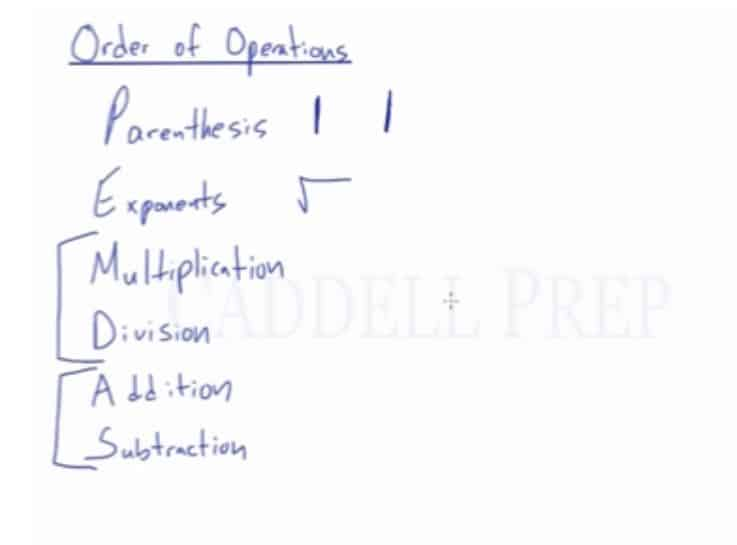In this video, we will be learning how to solve equations using order of operations.
Use PEMDAS:

Parenthesis
Exponent
Multiplication
Division
Subtraction

## Examples of Order of Operations

### Example 1$28 \div 4+3^{2}$

First, we will solve the number with exponent$28 \div 4+3^{2}= 28\div4+9$

Then, we will divide 28 by 4$28\div4+9= 7+9$

Now, we add the two numbers$7+9= 16$

Therefore, the answer$16$

### Example 2$(16-4)+3\times2$

First, we subtract the numbers inside the parenthesis

(16-4)+3\times2=(12)+3\times2

Then, we multiply 3 and 2
(12)+3\times2=12+6

Now, we add 12 and 6$12+6=18$

Therefore, the answer$18$

After you finish this lesson, view all of our Pre-Algebra and Algebra lessons and practice problems.

## Video-Lesson Transcript

In this video, we will be learning how to solve equations using the order of operations.

PEMDAS stands for:

Parenthesis
Exponent
Multiplication
Division
Subtraction

Multiplication and division can be interchanged. Whichever of the two comes first.

Same is true for addition and subtraction.

Square root has the same order as the exponent.

While the absolute Value Bars have the same order as the parenthesis.Let me give you an example.

We have$3 \mid 5 + (-4)\mid$

First, do the operation inside the absolute value bars first.

Some would think that it would be$= 3 \mid 5 + 4 \mid$

But it is not.

Let’s do$3 \mid 5 + (-4)\mid$

Here, we should compute the$\mid 5 + (-4) \mid$ first.

We will come up with$\mid 1 \mid$

So it will be$3 (1)$

And the answer is$3$

Notice that if we keep this$= 3 \mid 5 + 4 \mid$

The answer wouldn’t be the same.

Let’s move on to square roots.

We have$\sqrt{49} + \sqrt{36}$

We can’t just add these together.

We have to do the Exponent first.

So, it’s going to be$7 + 6$

Add these and we’ll have$13$

So there you have it.

Again, treat square root with the same order as exponent.

And absolute value bars with the same order as the parenthesis.